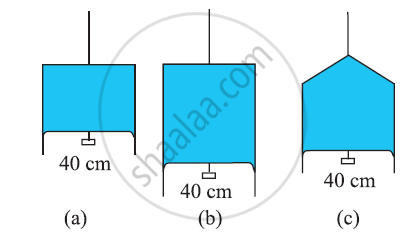CBSE (Science) Class 11CBSE
Share

# Figure (A) Shows a Thin Liquid Film Supporting a Small Weight = 4.5 × 10–2 N. What is the Weight Supported by a Film of the Same Liquid at the Same Temperature in Fig. (B) and (C)? Explain Your Answer Physically - CBSE (Science) Class 11 - Physics

#### Question

Figure  (a) shows a thin liquid film supporting a small weight = 4.5 × 10–2 N. What is the weight supported by a film of the same liquid at the same temperature in Fig. (b) and (c)? Explain your answer physically.#### Solution 1

Take case (a):

The length of the liquid film supported by the weight, l = 40 cm = 0.4 cm

The weight supported by the film, W = 4.5 × 10–2 N

A liquid film has two free surfaces.

∴Surface tension  = W/(2l)

= (4.5 xx 10^(-2))/(2xx0.4) = 5.625 xx 10^(-2) Mn^(-1)

In all the three figures, the liquid is the same. Temperature is also the same for each case. Hence, the surface tension in figure (b) and figure (c) is the same as in figure (a), i.e., 5.625 × 10–2 N m–1.

Since the length of the film in all the cases is 40 cm, the weight supported in each case is 4.5 × 10–2 N.

#### Solution 2

(a) Here, length of the film supporting the weight = 40 cm = 0.4 m. Total weight supported (or force) = 4.5 x 10-2 N.

Film has two free surfaces, Surface tension, S =4.5 x 10-2/2 x 0.4 =5.625 x 10-2 Nm-1
Since the liquid is same for all the cases (a), (b) and (c), and temperature is also same, therefore surface tension for cases (b) and (c) will also be the same = 5.625 x 10-2. In Fig. 7(b), 38(b) and (c), the length of the film supporting the weight is also the saihe as that of (a), hence the total weight supported in each case is 4.5 x 10-2 N.

Is there an error in this question or solution?

#### APPEARS IN

NCERT Solution for Physics Textbook for Class 11 (2018 to Current)
Chapter 10: Mechanical Properties of Fluids
Q: 18 | Page no. 269

#### Video TutorialsVIEW ALL 

Solution Figure (A) Shows a Thin Liquid Film Supporting a Small Weight = 4.5 × 10–2 N. What is the Weight Supported by a Film of the Same Liquid at the Same Temperature in Fig. (B) and (C)? Explain Your Answer Physically Concept: Surface Tension.
S